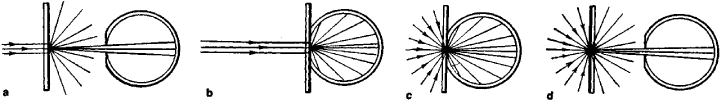# optical density

Also found in: Dictionary, Thesaurus, Medical, Legal, Financial, Acronyms, Wikipedia.

## optical density:

see refractionrefraction,
in physics, deflection of a wave on passing obliquely from one transparent medium into a second medium in which its speed is different, as the passage of a light ray from air into glass.
.

## Optical Density

a measure of the opacity of a layer of a substance to light rays. The optical density D is equal to the common logarithm of the ratio of the radiation flux F0 incident on the layer to the flux F that has been attenuated by absorption and scattering in passing through the layer: D = log (F0/F). In other words, the optical density is the logarithm of the reciprocal of the transmission coefficient of a layer of a substance: D = log (1/τ). In the definition of natural optical density, a concept that is also sometimes used, the common logarithm is replaced by the natural logarithm.Figure 1. Types of optical density of a layer of a medium classed according to the incident radiation’s geometry and the method used to measure the transmitted radiation flux, (a) The specular optical density Dǀǀ is determined by directing a parallel flux perpendicular to the layer and measuring only the part of the transmitted flux that retains the original direction, (b) To determine the totally diffuse optical density D, a parallel flux is directed perpendicular to the layer and the entire transmitted flux is measured, (c) and (d) Methods of measuring two types of doubly diffuse optical density D; the incident flux is uniformly diffuse. The difference DǀǀD is a measure of the light scattered in the layer under examination. In the sensitometric system used in the USSR the terms “regular,” “integral,” and “diffuse” are found instead of “specular,” “totally diffuse,” and “doubly diffuse,” respectively.

The concept of optical density was introduced by R. Bunsen. It is used to characterize the attenuation of optical radiation, or light, in layers and films of such various substances as dyes, solutions, stained glass, and milk glass, as well as in light filters and other optical products. The concept of optical density is especially widely used in the quantitative evaluation of developed photographic emulsions in both black-and-white and color photography; the methods used to measure it constitute the subject of densitometry. There are several types of optical density, depending on the nature of the incident radiation and the method of measurement of the transmitted radiation flux (Figure 1).

Optical density depends on the set of frequencies ν or wavelengths λ characterizing the initial flux; its value for the limiting case of one unique v is called the monochromatic optical density for this frequency. The specular monochromatic optical density (Figure 1,a) of a layer of a nondispersive medium is, not taking into account corrections for reflection from the front and rear boundaries of the layer, equal to 0.4343 kv l, where kv is the natural absorption coefficient of the medium and l is the thickness of the layer. Actually, kv l = kcl, which is the exponent in the equation of the Bouguer-Lambert-Beer law. If the scattering in the medium cannot be disregarded, kv is replaced by the natural attenuation coefficient. For a mixture of nonreacting substances or a series of media placed one on top of another, this type of optical density is additive—that is, it is equal to the sum of the optical densities of the individual substances or media. The same is true for specular polychromatic optical density, where the radiation has a complex spectral composition, in the case of media with nonselective absorption, that is, absorption independent of v. The specular polychromatic optical density of a series of media with selective absorption is less than the sum of the optical densities of the media.

### REFERENCES

Gorokhovskti, Iu. N., and T. M. Levenberg. Obshchaia sensitometriia: Teoriia i praktika. Moscow, 1963.
James, T., and G. Higgins. Osnovy teorii fotograficheskogo protsessa. Moscow, 1954. (Translated from English.)

L. N. KAPORSKII

## optical density

[′äp·tə·kəl ′den·səd·ē]
(optics)
The degree of opacity of a translucent medium expressed by log I0/ I, where I0 is the intensity of the incident ray, and I is the intensity of the transmitted ray. Abbreviated OD.
References in periodicals archive ?
Calibration curve for calcium, light filter No 7, cuvette--10 ml ml Optical density 0 0.
Optical density means were calculated based on the measurements taken in three different areas of each sample and on the fifth step of the step wedge (which corresponded to 5 mm).
Data presented are averages of triplicate determinations and their standard deviation of optical density and pH
Admitting that in the first stage the parallel dissolution of the lower-molecular weight fraction (1) and higher-molecular weight fraction (2) takes place, an increase in the total optical density of the solution should follow the relationship
These films are initially nearly transparent, and change their transmission color or optical density with exposure.
S]), the smoke optical density (OD) is calculated, which indicates the level of smoke obscuration.
Where the holes are more dense, there is more air than silicon, so the optical density of the object is reduced," Zhang said.
5,7,8) As reported earlier, (8) the authors have noticed changes in optical density (OD) on dry-processed films that patients left inside cars parked in the sun.
Eggs have also been found to increase macular pigment optical density (MPOD), which is also a counterindicator for AMD.
When blood was tested via enzyme-linked immunosorbent assay, the test patients had a mean optical density (OD; a measure of antibody levels) of 0.
Optical density measurements were done on tissue samples that had been immunochemically stained for 8-hydroxyguanosine, amyloid-[beta] 40, and amyloid-[beta] 42.

Site: Follow: Share:
Open / Close